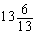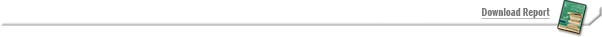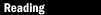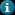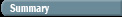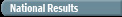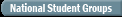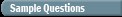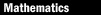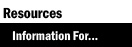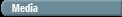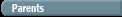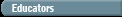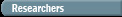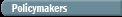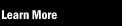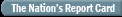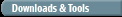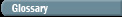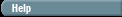Test Yourself Sample Questions: Mathematics

The following questions were included in the 2005 NAEP mathematics assessment at grade 12. As in the actual assessment, some of the questions are multiple-choice, and others are open-ended constructed-response items. Although students are asked to hand-write their responses, you can type yours in the space provided.

Question 1: If the digit in the tens place of 37,241 is increased by one and the digit in the thousands place is decreased by one, how has the number been changed?

 A. The number has been decreased by 990. B. The number has been decreased by 1,000. C. The number has been decreased by 1,010. D. The number has been increased by 10. E. The number has been increased by 1,010.

Question 2: If ƒ(x) = x2 + x and g(x) = 2x + 7, what is an expression
for ƒ(g(x)) ?

Question 3: The number of bacteria present in a laboratory sample
after t days can be represented by 500(2t). What is the initial number of bacteria present in this sample?

 A. 250 B. 500 C. 750 D. 1,000 E. 2,000

Question 4: Which of the right triangles below could NOT be a
30° - 60° - 90° triangle?

 A.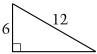B.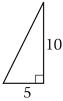C.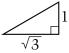D.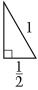E.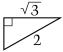Question 5: In a school fund-raiser, 10 students in class A sold an average (arithmetic mean) of 4 boxes of cookies. In class B, 15 students sold an average of 9 boxes. What was the average number of boxes of cookies sold by the 25 students?

 A.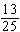B. 1 C.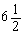D. 7 E.